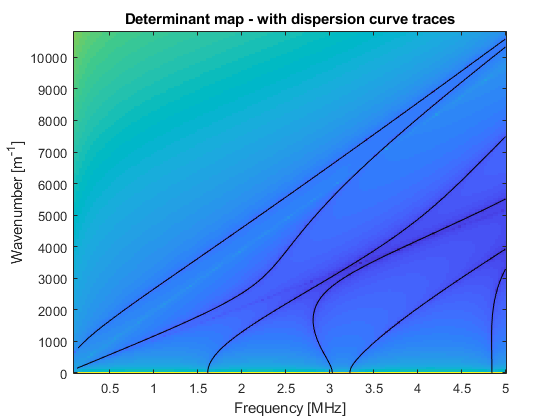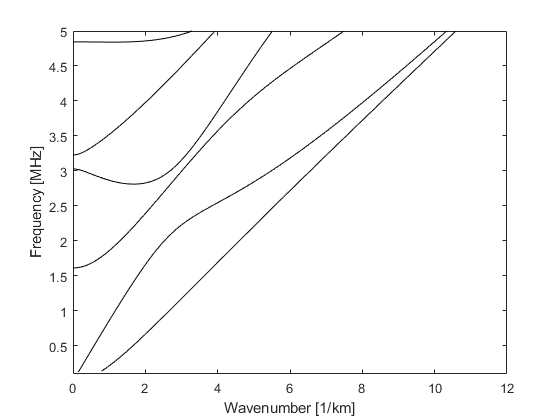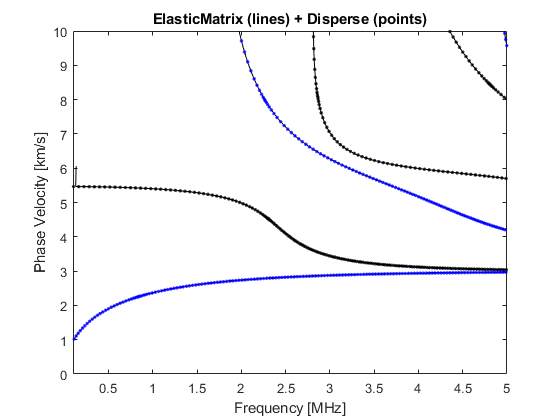# Example: Dispersion Curves - Titanium Plate

Dispersion curves describe the modal solutions of the multilayer structure and describe a wave-mode which propagates parallel to layer-interfaces independently of a bulk wave. Knowledge of these modes is essential in ultrasonic inspection.
The implemented dispersion curve algorithm follows the procedure given in . The ElasticMatrix software can calculate dispersion curves for simple layered structures (i.e., a plate in a vacuum or water). However, it is not robust for very-leaky cases, for example a plate embedded in an elastic medium. For these types of cases either Disperse , or other techniques based on the spectral-collocation method or semi-analytic finite element method are more appropriate. An example of the dispersion curves for a 1mm titanium plate in a vacuum is shown in here. The dispersion curves are plotted on a graph of frequency vs wavenumber and show the first three symmetric S and anti-symmetric A Lamb modes. The results from Disperse are also plotted and have excellent agreement.
 M. Lowe, Matrix techniques for modeling ultrasonic waves in multilayered media, IEEE Trans. Ultrason. Ferroelect. Freq. Contr. 42 (4) (1995) 525-542.
 B. Pavlakovic, M. Lowe, D. Alleyne, P. Cawley, Disperse: a general purpose program for creating dispersion curves, in: Review of progress in quantitative nondestructive evaluation, Springer, 1997.

## Initialize an ElasticMatrix Object

Initialize an ElasticMatrix object with a Medium object.
% A titanium plate:
my_medium = Medium('vacuum', 0, 'titanium', 0.001, 'vacuum', 1);
% Initialize the object:
my_model = ElasticMatrix(my_medium);

## Setting Calculation Parameters

Set a range of frequencies to calculate the dispersion curves. The dispersion curves are calculated in terms of frequency-wavenumber, the range of wavenumbers is automatically calculated based on the lowest sound-speed within the medium. Only the first and last frequency are used.
% Set a range of frequencies:
my_model.setFrequency(linspace(0.1e6, 5e6, 2)); % [Hz]

## Calculating Dispersion Curves

The dispersion curves are calculated using the .calculateDispersionCurve or .calculateDispersionCurveCoarse method. The .calculateDispersionCurve will attempt to trace the dispersion curves however, this does not always trace the modes correctly. If this is the case the .calculateDispersionCurveCoarse method can be used.
% Calculate the dispersion curves:
my_model.calculateDispersionCurves;... calculating dispersion curves ... ... finished calculating dispersion curves ...

## Plotting Dispersion Curves

The dispersion curves can be plotted using the .plotDispersionCurves method. Note: the algorithm will occasionally identify the critical angle as a dispersion curve.
figure_handles = my_model.plotDispersionCurves;% The data from a titanium plate calculation in Disperse is added:
disperse_data = importdata('disperseTitaniumPlateData.txt');
% Plot disperse points:
figure(figure_handles.fig2)
% S0
hold on
plot(disperse_data.data(:, 1), disperse_data.data(:, 2), 'k.')
% A0
plot(disperse_data.data(:, 3), disperse_data.data(:, 4), 'b.')
% S1
plot(disperse_data.data(:, 5), disperse_data.data(:, 6), 'k.')
% S2
plot(disperse_data.data(:, 7), disperse_data.data(:, 8), 'k.')
% A1
plot(disperse_data.data(:, 13), disperse_data.data(:, 14), 'b.')
% A2
plot(disperse_data.data(:, 15), disperse_data.data(:, 16), 'b.')
hold off
% labels
xlim([0.1 5])
ylim([0 10])
xlabel('Frequency [MHz]')
ylabel('Phase Velocity [km/s]')
box on
title('ElasticMatrix (lines) + Disperse (points)')